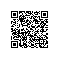# HDOJ 3466 Proud Merchants

Problem Description
Recently, iSea went to an ancient country. For such a long time, it was the most wealthy and powerful kingdom in the world. As a result, the people in this country are still very proud even if their nation hasn’t been so wealthy any more.
The merchants were the most typical, each of them only sold exactly one item, the price was Pi, but they would refuse to make a trade with you if your money were less than Qi, and iSea evaluated every item a value Vi.
If he had M units of money, what’s the maximum value iSea could get?

Input
There are several test cases in the input.

Each test case begin with two integers N, M (1 ≤ N ≤ 500, 1 ≤ M ≤ 5000), indicating the items’ number and the initial money.
Then N lines follow, each line contains three numbers Pi, Qi and Vi (1 ≤ Pi ≤ Qi ≤ 100, 1 ≤ Vi ≤ 1000), their meaning is in the description.

The input terminates by end of file marker.

Output
For each test case, output one integer, indicating maximum value iSea could get.

Sample Input
2 10
10 15 10
5 10 5
3 10
5 10 5
3 5 6
2 7 3

Sample Output
5
11

3 10 —-分别是商品件数和Money
5 10 5 —-A商品的价格，最低入手价，价值
3 5 6 —-B商品
2 7 3 —-C商品

 答案是11。正确的解法是先买A，再买B。这样就可以买到的价值是11，5+6=11。你会发现其实这个买的顺序有关系，因为你不可以先买B再
买A，我们先假设qi=pi，先把这个简单的问题解决。题目如下，其实就是把q省去了。


3 10
5 5 —-A商品
3 6 —-B商品
2 3 —-C商品

f(n，m)：花m元买n样东西实现的最大价值。对于任意的f(n，m)，都有下面这两种情况：

  情况一，你买了第n件商品，f(n, m)=f(n-1, m-pn)+vn，因为买了第n件商品，所以花费了pn元，也因此
得到了vn的价值。f(n, m)就等于第n件商品的价值+用m-pn的钱去买n-1件商品的价值。这样问题就规模就变小了。
情况二，你不买第n件商品，f(n, m)=f(n-1, m)，也就是说f(n, m)等于你用m元钱去买n-1件商品实现的最大价值。f(n,m)中n表示商品的件数，m表示钱，而f(0,3)表示没有商品，你有3块钱的情况下，你可以买到的价值，那当然是0咯；
在举个例子f(2,6)，表示有2件商品，你有6块钱，那你比较来判断你要不要买第二件商品，如果你买了第二件商品，那么
就是f(1, 6-3)+6=0+6=6；如果你不买第二件商品，那么就是f(1,6)=5。两种情况取大的，所以，你取6。其
实，以上表格就是根据f(n, m) = max(f(n-1, m-pn)+vn, f(n-1, m))来得到的，多设计几组数据练几下就融会贯通了。这
样第一步就算完成了，其实这就是0/1背包。


3 10
5 5 5 —-A商品
3 3 6 —-B商品
2 3 3 —-C商品

import java.sql.Array;
import java.util.Arrays;
import java.util.Comparator;
import java.util.Scanner;

public class Main{

public static void main(String[] args) {
Scanner sc = new Scanner(System.in);
chx[] a = new chx;
chx temp ;
while(sc.hasNext()){
int b[] = new int ;
int n = sc.nextInt();
int m = sc.nextInt();
//System.out.println(n+" "+ m);
for(int i=1;i<=n;i++){
a[i] = new chx();

a[i].p = sc.nextInt();
a[i].q = sc.nextInt();
a[i].v = sc.nextInt();
a[i].qp = a[i].q-a[i].p;
}

for(int i=1;i<n;i++){
for(int j=i+1;j<=n;j++){
if(a[i].qp>a[j].qp){
temp = a[i];
a[i] = a[j];
a[j] = temp;
}
}
}

for(int i=1;i<=n;i++){
for(int j=m;j>=a[i].q;j--){
b[j] = Math.max(b[j],b[j-a[i].p]+a[i].v);
}
}

System.out.println(b[m]);

}
}

}

class chx {
int p;
int q;
int v;
int qp;
public chx(){
p =0;
q =0;
v =0;
qp =0;
}

}使用钉钉扫一扫加入圈子
+ 订阅